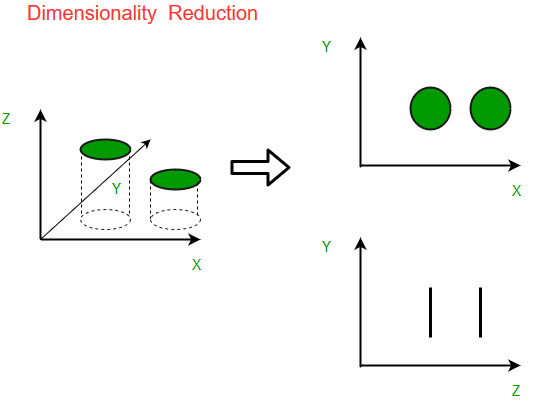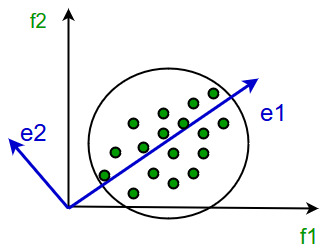Related Articles
Introduction to Dimensionality Reduction
• Difficulty Level : Easy
• Last Updated : 08 Feb, 2018

Machine Learning: As discussed in this article, machine learning is nothing but a field of study which allows computers to “learn” like humans without any need of explicit programming.

What is Predictive Modeling: Predictive modeling is a probabilistic process that allows us to forecast outcomes, on the basis of some predictors. These predictors are basically features that come into play when deciding the final result, i.e. the outcome of the model.

What is Dimensionality Reduction?

In machine learning classification problems, there are often too many factors on the basis of which the final classification is done. These factors are basically variables called features. The higher the number of features, the harder it gets to visualize the training set and then work on it. Sometimes, most of these features are correlated, and hence redundant. This is where dimensionality reduction algorithms come into play. Dimensionality reduction is the process of reducing the number of random variables under consideration, by obtaining a set of principal variables. It can be divided into feature selection and feature extraction.

Why is Dimensionality Reduction important in Machine Learning and Predictive Modeling?

An intuitive example of dimensionality reduction can be discussed through a simple e-mail classification problem, where we need to classify whether the e-mail is spam or not. This can involve a large number of features, such as whether or not the e-mail has a generic title, the content of the e-mail, whether the e-mail uses a template, etc. However, some of these features may overlap. In another condition, a classification problem that relies on both humidity and rainfall can be collapsed into just one underlying feature, since both of the aforementioned are correlated to a high degree. Hence, we can reduce the number of features in such problems. A 3-D classification problem can be hard to visualize, whereas a 2-D one can be mapped to a simple 2 dimensional space, and a 1-D problem to a simple line. The below figure illustrates this concept, where a 3-D feature space is split into two 1-D feature spaces, and later, if found to be correlated, the number of features can be reduced even further.Components of Dimensionality Reduction

There are two components of dimensionality reduction:

• Feature selection: In this, we try to find a subset of the original set of variables, or features, to get a smaller subset which can be used to model the problem. It usually involves three ways:
1. Filter
2. Wrapper
3. Embedded
• Feature extraction: This reduces the data in a high dimensional space to a lower dimension space, i.e. a space with lesser no. of dimensions.

Methods of Dimensionality Reduction

The various methods used for dimensionality reduction include:

• Principal Component Analysis (PCA)
• Linear Discriminant Analysis (LDA)
• Generalized Discriminant Analysis (GDA)

Dimensionality reduction may be both linear or non-linear, depending upon the method used. The prime linear method, called Principal Component Analysis, or PCA, is discussed below.

Principal Component Analysis

This method was introduced by Karl Pearson. It works on a condition that while the data in a higher dimensional space is mapped to data in a lower dimension space, the variance of the data in the lower dimensional space should be maximum.It involves the following steps:

• Construct the covariance matrix of the data.
• Compute the eigenvectors of this matrix.
• Eigenvectors corresponding to the largest eigenvalues are used to reconstruct a large fraction of variance of the original data.

Hence, we are left with a lesser number of eigenvectors, and there might have been some data loss in the process. But, the most important variances should be retained by the remaining eigenvectors.

• It helps in data compression, and hence reduced storage space.
• It reduces computation time.
• It also helps remove redundant features, if any.